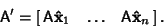## Basis

A (vector) basis is any Set ofLinearly Independent Vectors capable of generating an-dimensional Subspace of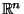. Given a Hyperplane defined by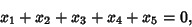a basis is found by solving for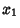in terms of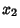,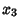,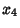, and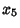. Carrying out this procedure,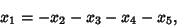so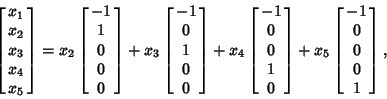and the above Vector form an (unnormalized) Basis. Given a Matrix A with an orthonormal basis, the Matrix corresponding to a new basis, expressed in terms of the original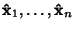is